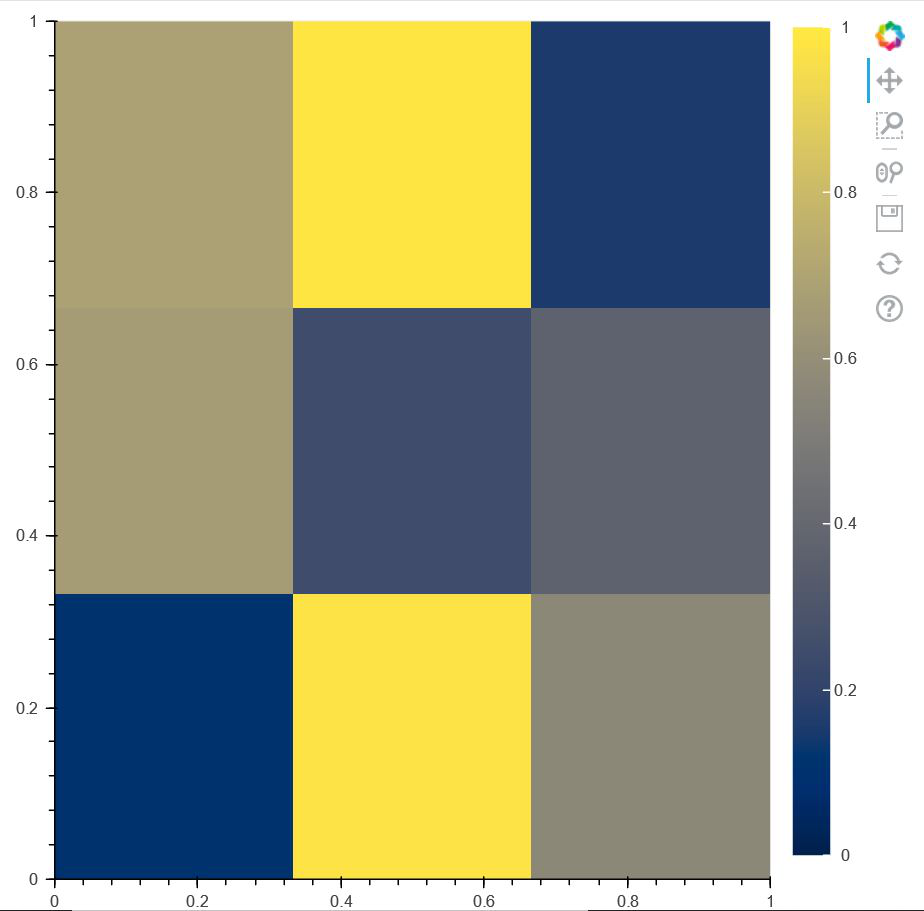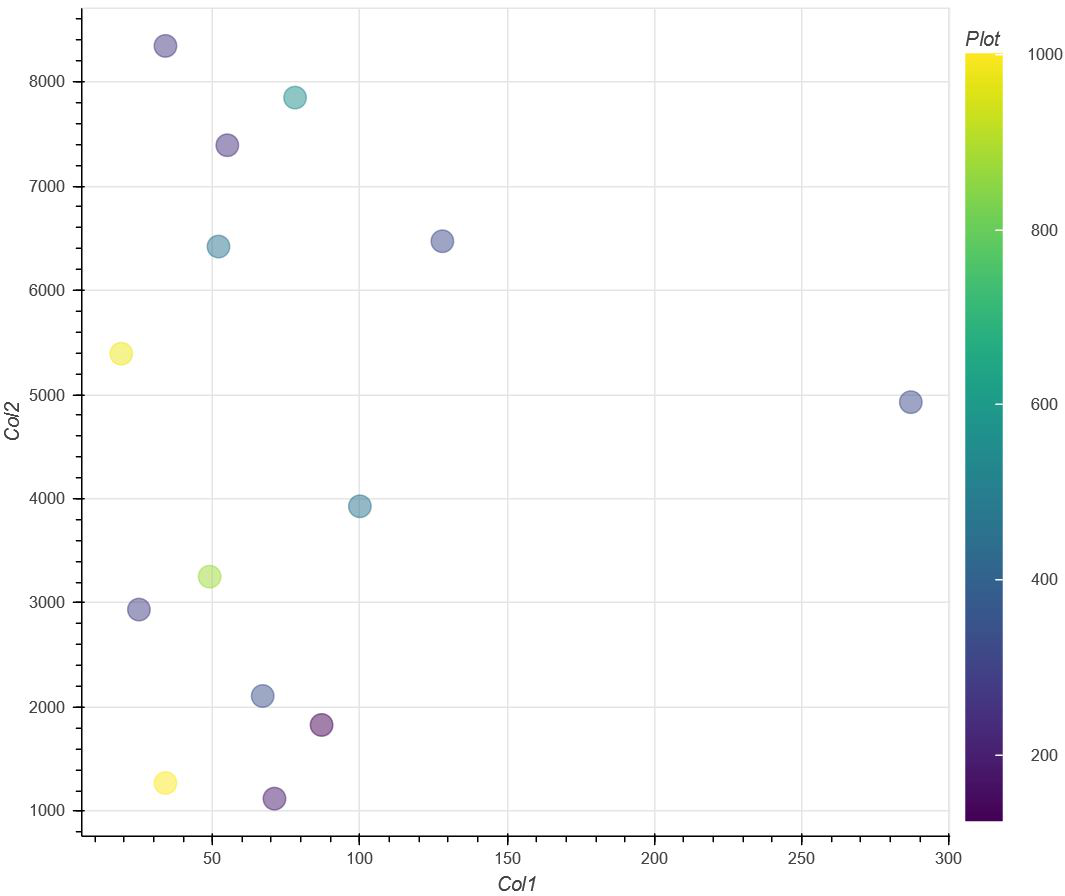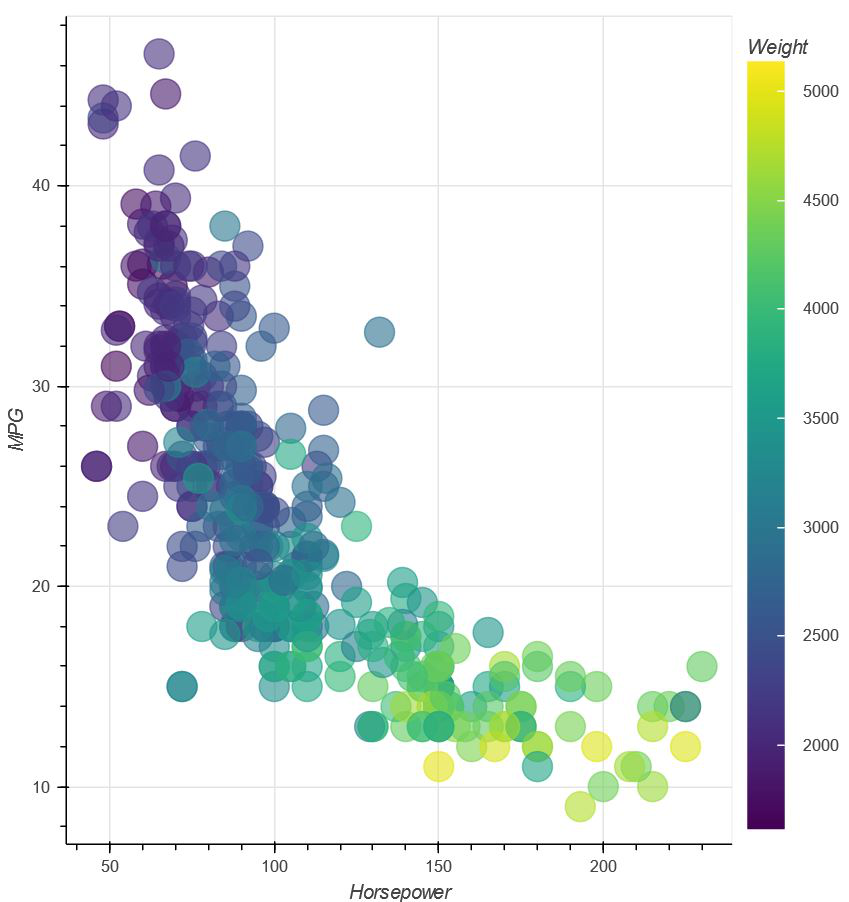GeeksforGeeks App
Open AppBrowser
Continue

# How to Add Color Bars in Bokeh?

Bokeh is one of the promising libraries of Python in recent times. It provides high performance and efficiency in data visualizations. One of the big advantages of bokeh is that we can get the output file in various formats such as HTML, notebooks etc. In this article, we will be learning about how to add Color Bars in Bokeh.

Now, there are various visualization interfaces that bokeh provides us such as bokeh.models, bokeh.plotting, bokeh.transform etc so that we can import various modules out of it and use them to create and define various properties in the plots.

One can use Google colab that has everything preinstalled in it but if we are using the local device, then it is very important to install bokeh in our device, else the functionalities won’t work. First, we need to open a command prompt on our desktop and go to the following directory where we want to install bokeh. Be sure, you already have node.js installed on your local device, else you can refer to this website for download. After going to the directory, write the following code in the command prompt.

`pip install bokeh`

Now, we can use any text editor for the implementation of the above concept.

Code:

## Python3

 `# importing numpy package``import` `numpy as np` `# importing figure and show for creating``# and showing plots from bokeh.plotting``# interface``from` `bokeh.plotting ``import` `figure, show` `# importing LinearColorMapper and ColorBar``# from bokeh.models to create color bars``from` `bokeh.models ``import` `LinearColorMapper, ColorBar` `# Creating a 2D array of scalar data to plot``d ``=` `[[``0.11023``,``0.97382``,``0.5634``],``     ``[``0.66382``,``0.2484``,``0.36524``],``     ``[``0.6845``,``0.9824``,``0.15498``]]` `# Using Linear color mapping, we are deciding``# palette color of the color bar and we are specifying``# low and high determining the range of values in color map``color ``=` `LinearColorMapper(palette ``=` `"Cividis256"``,``                          ``low ``=` `0``, high ``=` `1``)` `# Creating a figure where we define``# the x-Axis limits and y-Axis limits``# of the plot``plot ``=` `figure(x_range ``=` `(``0``, ``1``),``              ``y_range ``=` `(``0``,``1``))` `# Creating the image of the plot by``# specifying width and height of the inside plot``# along with color mapper and scalar data d``plot.image(image ``=` `[d], color_mapper ``=` `color,``           ``dh ``=` `[``1``], dw ``=` `[``1``], x ``=` `[``0``], y ``=` `[``0``])` `# Creating the color bar and setting the``# color bar position at (5,6)``cb ``=` `ColorBar(color_mapper ``=` `color, location ``=` `(``5``,``6``))` `# Adding the color bar to the right side``plot.add_layout(cb, ``'right'``)` `# Showing the above plot``show(plot)`

Output:Code Explain: After importing all the necessary packages in the code, we are creating a scalar data d where we are storing values ranging from 0 to 1. After that, using LinearColorMapper, we are defining the color of the palette and the range in the color-bar. Then, we are creating a figure where we are setting the X-Axis range and Y-Axis Range from 0 to 1. After that, we are plotting the above plot using .image() where we are defining the width and height of the plot along with the starting point of the plot as parameters. So, after fixing the position and labels of the color map, we are finally showing the above plot as an HTML file.

Example 2:

Instead of creating a heatmap, we can also create a simple plot and add a color bar to it. But for that, we need to create a dataframe in python. We need to install pandas in our local device if we are using it or if we use google colab , then we don’t need to install anything. Open a command prompt and write the following code :

`pip install pandas`

After the creation of the dataframe, we can process the data and add a color bar to it. The following implementation is shown below:

In this second example, we are not creating a heat map. Instead, we are creating a plot with points plotted on the graph in the shape of a circle with the color based on the colors from the color-bar. So, in order to achieve this, we need to create a set of data that is needed to be dataframed in order to get them in a tabular format. After getting the above implementation done, we will be using LinearColorMapper() in order to define the color and the range of the color-map. After that, we are creating a figure where we are defining the plot width and plot height along with X and Y-Axis Labels. Then, we are defining the shape of the points plotted i.e a circle and along with that we are using transform() inside which we are defining our color-map and the values that each of the points(x, y) will carry at that location. This means that at every point the color will be according to the value that it carries and the values are made from the ‘col0’ of the dataframe. Finally, we are showing our resultant plot.

Code:

## Python3

 `# importing linear color mapper and colorbar``# from bokeh.models``from` `bokeh.models ``import` `LinearColorMapper, ColorBar` `# importing transform from bokeh.transform``from` `bokeh.transform ``import` `transform` `# importing figure and show from``# bokeh.plotting``from` `bokeh.plotting ``import` `figure,show` `# importing pandas library``import` `pandas as pd` `# Creating a set of data in d``d``=``{``'Col0'``:[ ``190``, ``320``, ``270``, ``874``, ``459``, ``124``, ``546``,``           ``285``, ``341``, ``980``, ``1002``, ``453``, ``324``, ``245``],``   ``'Col1'``:[ ``71``, ``128``, ``34``, ``49``, ``52``, ``87``, ``78``, ``25``, ``67``,``           ``19``, ``34``, ``100``, ``287``, ``55``],``   ``'Col2'``:[ ``1123``, ``6471``, ``8345``, ``3253``, ``6420``, ``1830``,``           ``7849``, ``2937``, ``2108``, ``5392``, ``1273``, ``3928``, ``4927``, ``7392``]}` `# Converting the set of data into``# a dataframe` `df ``=` `pd.DataFrame(d)` `# Using linear color mapper, we are``# deciding the color of our color``# bar palette and also defining the``# lowest and highest values``color ``=` `LinearColorMapper(palette ``=` `'Viridis256'``,``                          ``low ``=` `df.Col0.``min``(),``                          ``high ``=` `df.Col0.``max``())` `# Creating a figure where we define``# its height and width along with its x-Axis``# label and Y-Axis Label``colorbar ``=` `figure(plot_width ``=` `750``, plot_height ``=` `600``,``                  ``x_axis_label ``=` `'Col1'``, y_axis_label ``=` `'Col2'``)` `# Plotting the points in the graph using``# circles where color of the circles will be``# according to their values in the color bar``# along with defined size and opacity``colorbar.circle(x ``=` `'Col1'``, y ``=` `'Col2'``,``                ``source ``=` `df, color ``=` `transform(``'Col0'``, color),``                ``size ``=` `15``, alpha ``=` `0.5``)` `# Defining various other features in the``# color bar such as its location in the``# plot along with its title``color_bar ``=` `ColorBar(color_mapper ``=` `color,``                     ``label_standoff ``=` `14``,``                     ``location ``=` `(``0``,``0``),``                     ``title ``=` `'Plot'``)` `# Defining the position of the color bar``colorbar.add_layout(color_bar, ``'right'``)` `# Showing the above implementation``show(colorbar)`

Output:Example 3:

In this example, we will be importing a dataset ‘autompg‘ from bokeh.sampledata.autompg.

## Python3

 `# importing figure and show from bokeh.plotting``# module``from` `bokeh.plotting ``import` `figure,show` `# importing autompg from bokeh.sampledata.autompg``from` `bokeh.sampledata.autompg ``import` `autompg` `# importing LinearColorMapper and ColorBar``# from bokeh.models``from` `bokeh.models ``import` `LinearColorMapper, ColorBar` `# importing transform from bokeh.transform``from` `bokeh.transform ``import` `transform``print``(autompg)` `# Defining the palette color along with``# the max and min values of the color bar``color_mapper ``=` `LinearColorMapper(palette ``=` `"Viridis256"``,``                                 ``low ``=` `autompg.weight.``min``(),``                                 ``high ``=` `autompg.weight.``max``())` `# Creating an empty figure where we define the``# X-Axis label, Y-Axis Label``p ``=` `figure(x_axis_label ``=` `'Horsepower'``,``           ``y_axis_label ``=` `'MPG'``)` `# Drawing circular plots in the graph with``# color based on the value from the``# color-mapper``p.circle(x ``=` `'hp'``, y ``=` `'mpg'``, color ``=` `transform(``'weight'``,``                                                ``color_mapper),``         ``size ``=` `20``, alpha ``=` `0.6``, source ``=` `autompg)` `# Defining properties of color mapper``color_bar ``=` `ColorBar(color_mapper ``=` `color_mapper,``                     ``label_standoff ``=` `12``,``                     ``location ``=` `(``0``,``0``),``                     ``title ``=` `'Weight'``)` `# Defining position of the color-mapper``p.add_layout(color_bar, ``'right'``)` `# Showing the plot``show(p)`

Output:In the third example, we are importing a dataset using bokeh.sampledata.autompg and since it is already in tabular format, we don’t need to use dataframing. Then we are following the same steps to that of Example 2 and showing the above plot.

My Personal Notes arrow_drop_up